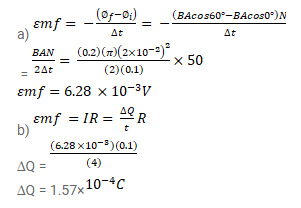# A circular coil of radius 2.00 cm and 50 turns.

Question:

A circular coil of radius $2.00 \mathrm{~cm}$ and 50 turns. A uniform magnetic field $B=0.200 \mathrm{~T}$ exists in the space in a direction parallel to the axis of the loop. The coil is now rotated about a diameter through an angle of $60^{\circ}$. The operation takes $0.100 \mathrm{~s}$.

(a) Find the average emf induced in the coil.

(b) If the coil is closed one (with the two ends joined together) and has a resistance of $4.00 \Omega$, calculate the net crossing a cross-section of the wire of the coil.

Solution: